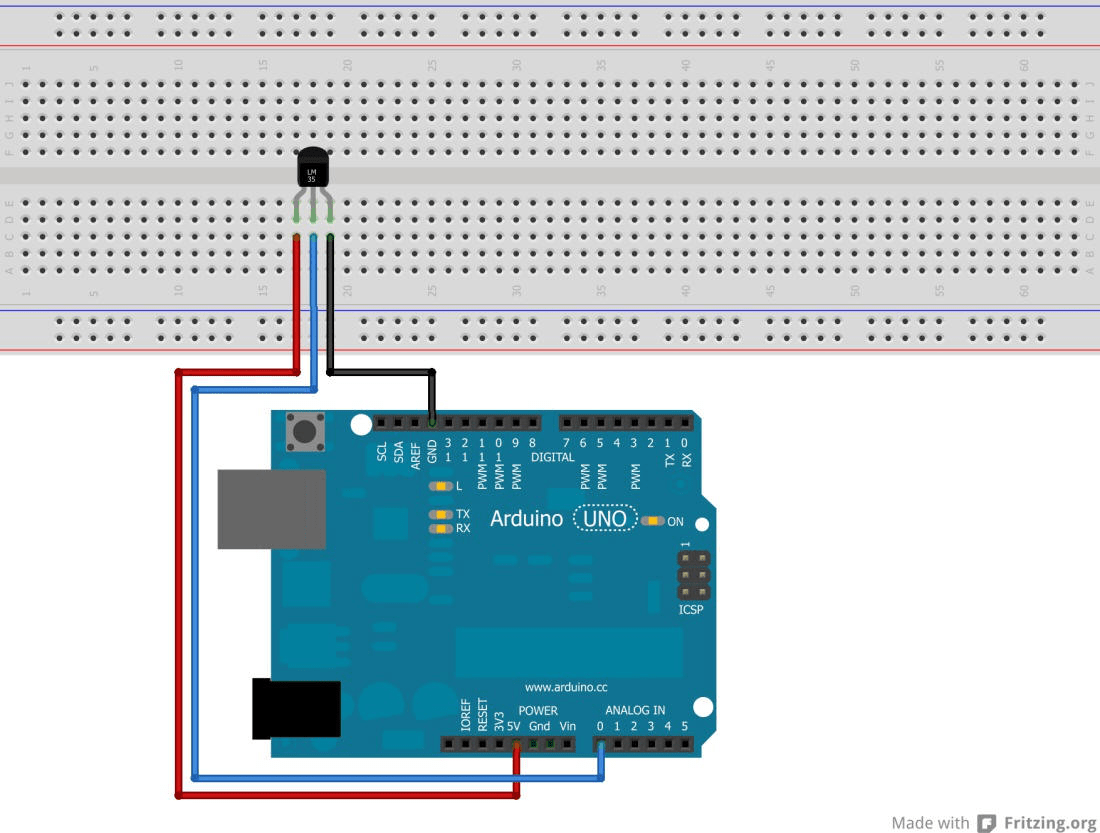# No. 10 – Temperature measurement

Task: Read out the temperature with theTMP36 sensor and display the temperature on the serial monitor.

Required equipment: Arduino / Breadboard / cables / temperature sensor TMP36 / external power-supply

The sensor has three terminals. 5V, GND, and the pin for the temperature signal. On this pin, the sensor puts out a voltage between 0 and 2.0 volts.
0V = -50 ° C and 2.0V = 150 ° C.
The sensor is supposed to be quite precise (±2°C) between -40°C and 150°C, according to the manufacturing. The voltage on this pin must be read out by the microcontroller board and then has to be converted into a temperature value.

– CAUTION: If the sensor is connected incorrectly it gets destroyed.

– Use a external power supply for more sensor accuracy (as possible 9V battery or 9V power supply).

Setup:Code:

int TMP36 = A0; //The sensor should be connected to the analog Pin A0. We will call the pin “TMP36” from //now on

int temperature = 0; //Under the variable “temperature” we will later on save the temperature value

int temp; //To get good values we have to read out some values to get the mean value out of them. The //square brackets “” create ten different variables at one time:„temp“, „temp“, „temp“, …till… //„temp“.So with this spelling  we will save some space.

int time= 20; //The value after “time” sets the distance between each measurement.

void setup() {

Serial.begin(9600); //In the setup we are going to start the serial communication, to see the temperature //values on the serial monitor. The microcontroller will be sending values to the computer. The serial //monitor can be started at “Tools” in the arduino software.

}

void loop() { //main part

temp = map(analogRead(TMP36), 0, 410, -50, 150);

delay(time);

temp = map(analogRead(TMP36), 0, 410, -50, 150);

delay(time);

temp = map(analogRead(TMP36), 0, 410, -50, 150);

delay(time);

temp = map(analogRead(TMP36), 0, 410, -50, 150);

delay(time);

temp = map(analogRead(TMP36), 0, 410, -50, 150);

delay(time);

temp = map(analogRead(TMP36), 0, 410, -50, 150);

delay(time);

temp = map(analogRead(TMP36), 0, 410, -50, 150);

delay(time);

temp = map(analogRead(TMP36), 0, 410, -50, 150);

delay(time);

temp = map(analogRead(TMP36), 0, 410, -50, 150);

delay(time);

temp = map(analogRead(TMP36), 0, 410, -50, 150); //Until here the temperature gets read out ten times. //In between there is always a little //break. We will take a closer look on the used command:

//temp – The name of the first variable.

//“map(a,b,c,d,e)” – This is a “Map command”. With this command it is possible to change a read out value //(a) from one region between (b) and (c) into a //region between (d) and (e).In our case this means: The //sensor value gets read out right in the map //command “analogRead(TMP36)”. The value should be between 0 //and 410 (= values between 0V and 2V at the analog port). The sensor outputs this voltage values if it //measures temperature between -50°C and 150°C. With the “map command” //these values get converted into //degree values between -50°C and 150°C.

temperature=(temp+temp+temp+temp+temp+temp+temp+temp+temp+temp)/10; //Everything in one row! In this line, all ten values get summarized and get divided by ten. This average //value gets saved under

//“temperature”.

Serial.print(temperature); //Now the temperature values gets send to the computer and can be looked up on //the serial monitor.

Serial.println(„degree“);

}

Extension of the sketch:

If the temperature reaches 30°C, a noise from the piezo speaker appears.

int TMP36 = A0;

int temperature = 0;

int temp;

int time= 20;

int piezo=5; //Piezo speaker on pin 5

void setup() {

Serial.begin(9600);

pinMode (piezo, OUTPUT); //Pin 5 is an output

}

void loop() {

temp = map(analogRead(TMP36), 0, 410, -50, 150);

delay(time);

temp = map(analogRead(TMP36), 0, 410, -50, 150);

//…

temp = map(analogRead(TMP36), 0, 410, -50, 150);

temperature=(temp+temp+temp+temp+temp+temp+temp+temp+temp+temp)/10;

Serial.print(temperature);

Serial.println(degrees);

if (temperature>=30) //If the temperature reaches 30°C or more….

{

digitalWrite(piezo,HIGH); //…the piezo speakers beeps..

}

else

{

digitalWrite(piezo,LOW); //…it is quiet.

}

}